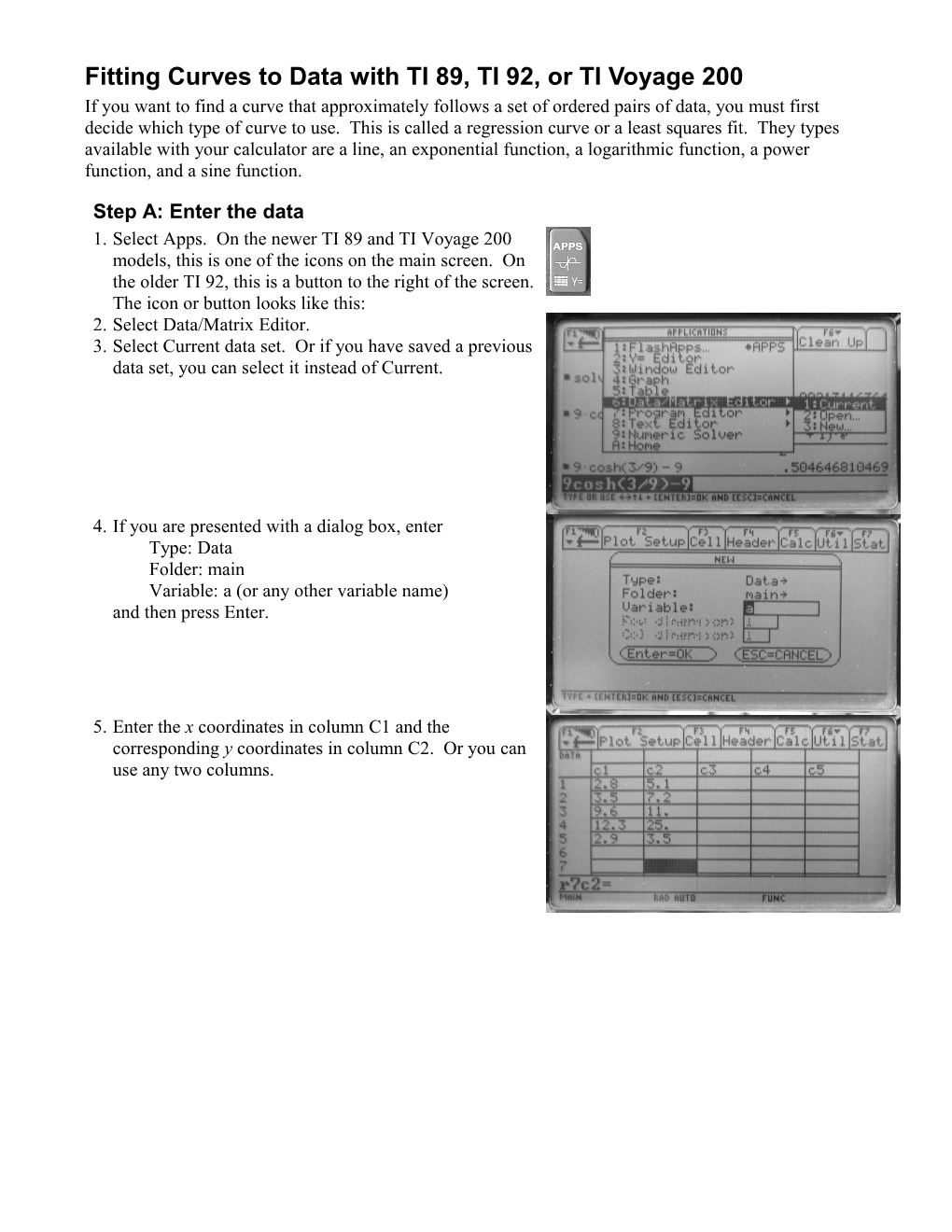Fitting Curves to Data with TI 89, TI 92, Or TI Voyage 200Fitting Curves to Data with TI 89, TI 92, or TI Voyage 200

If you want to find a curve that approximately follows a set of ordered pairs of data, you must first decide which type of curve to use. This is called a regression curve or a least squares fit. They types available with your calculator are a line, an exponential function, a logarithmic function, a power function, and a sine function.

Step A: Enter the data

1.Select Apps. On the newer TI 89 and TI Voyage 200 models, this is one of the icons on the main screen. On the older TI 92, this is a button to the right of the screen. The icon or button looks like this: /
2.Select Data/Matrix Editor.
3.Select Current data set. Or if you have saved a previous data set, you can select it instead of Current. /4.If you are presented with a dialog box, enter
Type: Data
Folder: main
Variable: a (or any other variable name)
and then press Enter. /5.Enter the x coordinates in column C1 and the corresponding y coordinates in column C2. Or you can use any two columns. /Step B: Find an equation for the curve

1.While still in the Data/Matrix Editor screen, select F5(Calc).
2.In the dialog box, select the Calculation Type:
LinReg — fit a line to the data, y = a x + b
ExpReg — fit an exponential function to the data,
y = a bx
LnReg — fit a logarithmic function to the data,
y = a + b ln(x)
y = a x2 + b x + c
CubicReg — fit a cubic function to the data,
y = a x3 + b x2 + c x + d
QuartReg — fit a quartic to the data,
y = a x4 + b x3 + c x2 + d x + e
SinReg — fit a sine function to the data,
y = a sin(b x – c) + d
Logistic — fit a logistic function to the data,
y = a/(1 + b ec x) + d /3.Enter the columns in which the x and y data reside, such as C1 and C2.
4.If you want to do any calculations with your curve or plot your curve, then select a location in the Y= screen where you want to store the equation. For example,
Store RegEQ to: y1(x)
will store the regression equation in the y1 line of the Y= screen. You can later use y1 in calculations or graphs. For example, if you later enter y1(3.7) in the Home screen, this will give you the value of the regression equation at x = 3.7.
5.Press Enter to get the regression equation. A pop-up dialog box will display the values of the parameters, a, b, etc., and the values of the correlation coefficient and R2. These two numbers are between 0 and 1. Values closer to 1 indicate that the regression equation fits the data well. You will learn about this in a statistics course. /Step C: Plot the graph

If you want to plot the graph of the data points and the regression curve, then you will need to store the regression equation in one of y1, y2, etc. See Step B.4 above. You can plot the equation in y1, y2, etc., in the same way that you plot any equation. Follow the steps below to plot the data points.
1.Select the Apps icon or button, as in Step A.1.
2.Select Data/Matrix Editor.
3.Select Current data set. Or if you have saved a previous data set, you can select it instead of Current.
4.In the spreadsheet-type screen where you entered the data into columns C1 and C2, select F2(Plot Setup).
5.In the dialog box that pops up, select F1(Define).
6.Another dialog box will be displayed. Enter the following:
Plot Type: Scatter
Mark: Box (or select any other symbol to represent the data points on the graph)
x: C1 (or whichever column contains the x coordinates)
y: C2 (or whichever column contains the x coordinates)
Press Enter to create the plot of data points and return to the Plot Setup screen and enter again to return to the data entry screen. /7.To view your plot, go to the GRAPH window (press diamond and then GRAPH)
8.Select F2(Zoom) and then ZoomData to display a view window that includes all of the data points. Your regression curve should appear in the graph along with the data points. /
9.In the Y= screen, the regression equation will be on the y1 line and above that, the data plot will be represented by the Plot 1 line. The check mark in the left column indicates that these two plots are to be displayed in the graph. Use F4 to toggle the check marks on and off. Delete the y1 and Plot 1 lines when in the Y= screen when the plots are no longer needed. If the entries in columns C1 and C2 are deleted but Plot 1 is not deleted, then the GRAPH window will display a cryptic error message. So, be certain to delete Plot 1 after clearing C1 and C2. /